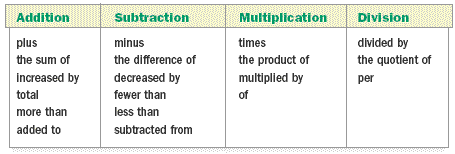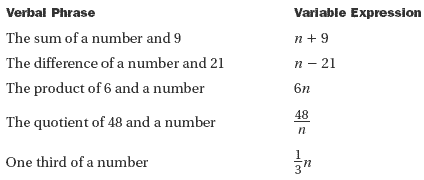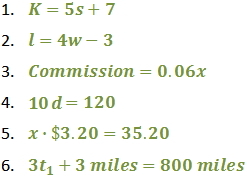Lesson - Translate Phrases to Mathematical Expressions

Translate Phrases to Mathematical Expressions

Many words and phrases suggest mathematical operations . The following common words and phrases indicate addition, subtraction, multiplication, and division.Verbal phrases can be translated into variable expressions. Some examples are below.Whenever possible, select a single variable to represent an unknown quantity. Then express related quantities in terms of the first variable selected.

Examples

For each relationship, select a variable to represent one quantity and state what that variable represents. Then express the second quantity in terms of the variable selected.

a) The Kings scored 7 more points than the Rangers.

Let r = number of points scored by the Rangers
Let r + 7 = number of points scored by the Kings

b) Bob and Marc share \$65.

Let a = how much Bob receives.
Let 65 - a = amount Marc receives.

Practice

Write each statement as an algebraic expression.

1) Kim has 7 more than 5 times the amount Sylvia has.

2) The length of a rectangle is 3 feet less than 4 times its width.

3) Write an expression to represent how much a realtor will earn at a 6% commission on a house that costs x dollars.

Write each problem below as an equation.

4) The number of cents in d dimes is 120.

5) The cost of x gallons of gasoline at \$3.20 per gallon is \$35.20.

6) One train travels 3 miles more than twice the distance another train travels. The total distance traveled by both trains is 800 miles.